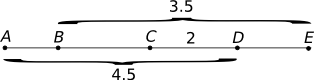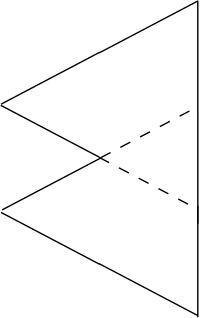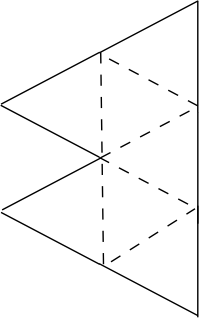#Solutions for Practice Test 1, The Official SAT Study Guide, Section 7

Math Lair Home > Test Preparation > Solutions for Practice Test 1, The Official SAT Study Guide, Section 7
SAT Practice Test Solutions:
2014–15 SAT Practice Test
2013–14 SAT Practice Test
The Official SAT Study Guide, second edition
• Practice Test 1: Sections 3, 7, 8.
• Practice Test 2: Sections 2, 5, 8.
• Practice Test 3: Sections 2, 5, 8.
• Practice Test 4: Sections 3, 6, 9.
• Practice Test 5: Sections 2, 4, 8.
• Practice Test 6: Sections 2, 4, 8.
• Practice Test 7: Sections 3, 7, 9.
• Practice Test 8: Sections 3, 7, 9.
• Practice Test 9: Sections 2, 5, 8.
• Practice Test 10: Sections 2, 5, 8.
SAT Math Tips

Here are solutions for section 7 of the first practice test in The Official SAT Study Guide, second edition, found on pages 413–418. The following solutions illustrate faster, less formal methods that may work better than formal methods on a fast-paced test such as the SAT. To learn more about these methods, see my e-book Succeeding in SAT Mathor the SAT math tips page.

• Estimate the answer: Just eyeballing the pictograph, it shows at least a dozen (probably slightly more) homes built between 1961 and 1990. So, the number of new homes will be slightly greater than 12 × 2000 = 24,000.
• Look at the answer choices: The only answer choice greater than 24,000 is (E) 28,000. Select that answer.
• Estimate the answer: The desired angle looks slightly greater than a right angle. Perhaps the answer is around 100°.
• Look at the answer choices: (B) 100° is one of the answer choices, but (A) 90° and (C) 110° look close. We can eliminate (D) and (E).
• Draw a diagram: The diagram is given, but we can fill in some angles. The other two angles in the triangle are opposite 35° and 45° angles, so their measurements must be 35° and 45°, respectively. Therefore, the value of w, the third angle in the triangle, must be 180 − 35 − 45 = 100 (since "The sum of the measures in degrees of the angles of a triangle is 180.") Therefore, the answer is (B) 100.
• Consider another problem:
• What if every table at the restaurant seated 4 people? Then the restaurant would seat 4 × 19 = 76 people.
• Going back to the original problem, the restaurant seats 84 people, which is 8 people more than in our alternate problem. Therefore, we need to add 1 chair to 8 tables in order to seat 84. Therefore, the answer is (E) 8.
• Look at the answer choices: All of the answer choices have a "4" factored out of them. Knowing this, we can do the same, so it's more likely that our answer will look like one of the answer choices.
• Evaluate the expression:
am² + am + a
= a(m² + m + 1)
= 4(m² + m + 1)
This expression matches (D) 4(m² + m + 1). Select that answer.
• Estimate the answer: The area of the square is 2 × 2 = 4. The area of the triangle is ¼ the area of the square, or 1. The area of the shaded bit must be less than 1, maybe around 0.8 or so.
• Look at the answer choices: The only choice less than 1 is (A) π⁄4. (Don't forget that π is simply a number slightly larger than 3, so you can tell that the other choices are greater than 1). Select (A) π⁄4.
1. Solution 1:
• Read the question and understand what it is asking: You are looking for a line perpendicular to the one given; in other words, one that intersects that line at right angles.
• Draw a diagram: The diagram is already given, but it is helpful to draw a line perpendicular to the given one. (Tip: One possibility is to fold your page over without creasing it, positioning it so that the edge of the page forms a right angle with the given line.)
• Estimate the answer: The line you just drew has a positive slope, and it is fairly steep, so the slope is going to be around 2 or 3 or 4. Therefore, the correct equation will be similar to y = 2x + something or 3x + something, etc.
• Look at the answer choices: The only equation having slope greater than 1 is (C) y = 3x + 6. Select that answer.
Solution 2:
• Draw a diagram: On your graphing calculator, graph x + 3y = 12.
• Guess and check: Graph each answer choice and see which one is perpendicular to the first line. You should find the answer is (C) y = 3x − 6.
2. Solution 1:
• Draw a diagram: Draw a diagram, perhaps similar to the following:If it helps, you may want to draw multiple figures.
• In the above diagram, take a look at points that I've labelled A and B. If you travel from A to B along the two sides of length 5, the distance is 10. Since the shortest distance between two points is a straight line, the distance from A to B along the third side of the triangle must be less than 10 (this is called the triangle inequality). Therefore, the length of the third side cannot be (E) 10.
Solution 2: I don't recommend this solution unless you have a programmable calculator and you've programmed Heron's formula on it (too time-consuming otherwise), but if so, then you can use guess and check. For each answer choice, use Heron's formula to evaluate the area of the resulting triangle. You will find that, for (E), the area is 0, so that indicates something is amiss. Select (E) 10.
3. Solution 1:
• Estimate the answer: 28,000 is 1% of 2.8 million. So, Candidate I probably got around 51% of the votes.
• Look at the answer choices: 51% is one of the possible answers, but there are others nearby. Probably (E) 55% could be eliminated, though.
• Take advantage of question order: This is the last multiple-choice question in the section, so it is likely to be quite hard. So, any "obvious" answer obtained without calculating is probably wrong. Eliminate (D) 51%.
• Calculate the answer:
• Let x represent the number of votes cast for Candidate I.
• Then, x − 28,000 is the number of votes cast for Candidate II.
x + x − 28000 = 2800000
2x = 2828000
x = 1414000
The percentage of votes cast for Candidate I is 1414000/2800000 × 100% = 50.5%. Therefore, the answer is (C) 50.5%.
Solution 2:
• Read the question carefully and determine what it is asking: You are looking for the percentage of votes cast for Candidate I if Candidate I received 28,000 more votes, or 1% more, than Candidiate II.
• Guess and check: For each answer, determine the percentage of votes cast for Candidate II, and see if the difference is 1%. Looking at answer (C), if Candidate I has 50.5%, Candidate II has 49.5%, and the difference is 1%. Therefore, the answer is (C) 50.5%.
4. The easiest way to solve this equation is by inspection. √2 · 9 = √18, so p = 9. Enter 9.
5. When 1.783 is rounded to the nearest whole number, the result is 2.
When 1.783 is rounded to the nearest tenth, the result is 1.8.
The required answer is 2 − 1.8 = .2.
6. If the probability is 2⁄5 that she will pick a brown towel, then 2⁄5 of the towels in the closet are brown. We know there are 6 brown towels. To get the total number of towels in the closet, divide the number of brown towels by the fraction of brown towels in the cupboard:
6 ÷ (2⁄5) = 15
Enter 15 as the answer.
• Read the question and understand what it is asking: The fact that the question asks for "one possible value" suggests that there is a range of values for BC, and we just need to find one.
• Draw a diagram:From the diagram, it's easy to see that BC + DE must be equal to 1.5.
• Try a special case: Try a reasonable-looking number that's easy to fill in on the answer sheet and see if it solves the problem. Could BC = 1? Yes, because then DE could be 0.5 and AB 1.5. Enter 1 as the answer.
7. One way of looking at the problem is as follows: For every five days, the number of extra days on which it rained increases by 1. After 6 × 5 = 30 days, the number of extra days on which it rained will be 6 greater. Enter 6 as the answer.
8. The difference between the third term and the sixth term is 77 − 17 = 60. Since:
sixth term = third term + 3 common differences
or (sixth term − third term) = 3 common differences
the common difference is 60 ÷ 3 = 20. So, the eighth term is 77 + 20 + 20 = 117.
9. |x − 3| = ½
x − 3 = ½ or x − 3 = −½
x = 3.5 or x = 2.5
We are asked for the least value of x, which is 2.5.
10. Guess and check: Z looks like it has to be pretty small compared with the other numbers. Say that Z = 0. Then W = 5, Y = 4, and X = 9. This makes 5940, which fits. Enter 5940.
11. Draw a diagram: A diagram is already given, but it isn't drawn to scale. Draw a diagram that is roughly to scale:If you add a few more lines to the diagram, you can see that it can be divided into several equilateral triangles of side length 10:The border of the shape is equal to 9 sides of the equilateral triangles. Since each side is of length 10, the length of the border is 9 × 10 = 90.
12. First, find where g(x) = 0:
k(x + 3)(x − 3) = 0
x = −3 or x = 3
If g(a − 1.2) = 0, then a − 1.2 = −3 or 3. If a > 0, only the positive possibility will work out:
a − 1.2 = 3
a = 4.2

Enter 4.2 as the answer.